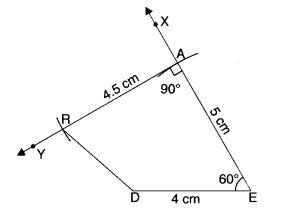NCERT Solutions for Class 8 Maths Chapter 4 Practical Geometry Ex 4.4 are part of NCERT Solutions for Class 8 Maths. Here we have given NCERT Solutions for Class 8 Maths Chapter 4 Practical Geometry Ex 4.4.

 Board CBSE Textbook NCERT Class Class 8 Subject Maths Chapter Chapter 4 Chapter Name Practical Geometry Exercise Ex 4.4 Number of Questions Solved 1 Category NCERT Solutions

## NCERT Solutions for Class 8 Maths Chapter 4 Practical Geometry Ex 4.4

Question 1.
DE = 4 cm
EA = 5 cm
AR = 4.5 cm
∠E = 60°
∠A = 90°

TR = 3.5 cm
RU = 3 cm
UE = 4 cm
∠R = 75°
∠U= 120°
Solution.
(i) Steps of Construction

1. Draw DE = 4 cm.
2. At E, draw ray EX such that ∠DEX = 60°.
3. From ray EX, cut EA = 5 cm.4. At A, draw ray AY such that ∠EAY = 90°.
5. Cut AR = 4.5 cm from ray AY.
6. Join RD.

Then, DEAR is the required quadrilateral.

(ii) Steps of Construction

1. Draw TR = 3.5 cm.
2. At R, draw ray RX such that ∠TRX = 75°.3. Cut RU = 3 cm from ray RX.
4. At U, draw ray UY such that ∠RUY = 120°.
5. Cut UE = 4 cm from ray UY.
6. Join ET.

Then, TRUE is the required quadrilateral.

We hope the NCERT Solutions for Class 8 Maths Chapter 4 Practical Geometry Ex 4.4 help you. If you have any query regarding NCERT Solutions for Class 8 Maths Chapter 4 Practical Geometry Ex 4.4, drop a comment below and we will get back to you at the earliest.